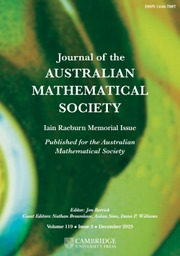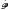Home
Hostname: page-component-568f69f84b-8fhp6 Total loading time: 0.257 Render date: 2021-09-16T10:03:33.896Z Has data issue: true Feature Flags: { "shouldUseShareProductTool": true, "shouldUseHypothesis": true, "isUnsiloEnabled": true, "metricsAbstractViews": false, "figures": true, "newCiteModal": false, "newCitedByModal": true, "newEcommerce": true, "newUsageEvents": true }Journal of the Australian Mathematical Society

# On continuation of quasi-analytic solutions of partial differential equations to compact convex sets

Published online by Cambridge University Press:  09 April 2009

## Abstract

HTML view is not available for this content. However, as you have access to this content, a full PDF is available via the ‘Save PDF’ action button.

In the early 70s A. Kaneko studied the problem of continuation of regular solutions of systems of linear partial differential equations with constant coefficients to compact convex sets. We show here that the conditions be obtained for real analytic solutions also hold in the quasi-analytic case. In particular we show that every quasi-analytic solution of the system p(D)u = 0 defined outside a compact convex subset K or Rn can be continued as a quasi-analytic solution to K if and only if the system is determined and the-module Ext1(Coker p′,) has no elliptic component; hereis the ring of polynomials in n variables, p is a matrix with elements fromand p′ is the transposed matrix. In the scalar case, i.e. when p is a single polynomial, these conditions mean that p has no elliptic factor.

## MSC classification

Type
Research Article
Information
Journal of the Australian Mathematical Society , December 1985 , pp. 306 - 316

## References

Björk, J. E., Rings of differential operators (North-Holland, Amsterdam, 1979).Google Scholar
Bochner, S., ‘Partial differential equations and analytic continuation’, Proc. Nat. Acad. Sci. 38 (1952), 227230.CrossRefGoogle Scholar
Boman, J., ‘On the propagation of analyticity of solutions of differential equations with constant coefficients’, Ark. Mat. 5 (1964), 271279.CrossRefGoogle Scholar
Ehrenpreis, L., ‘A new proof and an extension of Hartogs' theorem’, Bull. Amer. Math. Soc. 67 (1961), 507509.CrossRefGoogle Scholar
Grušin, V. V., ‘On solutions with isolated singularities for partial differential equations with constant coefficients’, Trudy Moskov. Mat. Obšč. 15 (1966), 262278 (in russian).Google Scholar
Hartogs, F., ‘Einige Folgerungen aus der Cauchyschen Integralformel bei Funktionen mehrer Veränderlichen’, Sitzb. Münchener Acad. 36 (1906), 223.Google Scholar
Hörmander, L., ‘Between distributions and hyperfunctions’, (Proc. Colloq. L. Schwartz, 1983, to appear in Astérisque).Google Scholar
Kaneko, A., ‘On continuation of regular solutions of partial differential equations to compact convex sets’, J. Fac. Univ. Tokyo, Sect IA, 17 (1970), 567580.Google Scholar
Kaneko, A., ‘On continuation of regular solutions of partial differential equations to compact convex sets’, J. Fac. Univ. Tokyo, Sect IA 18 (1972), 415433.Google Scholar
Kaneko, A., ‘Note on continuation of real analytic solutions of partial differential equations with constant coefficients’, Proc. Japan Acad. 51 (1975), 262264.CrossRefGoogle Scholar
Kaneko, A., ‘Prolongement des solutions analytiques réelles d'équations aux dérivées partielles á coefficients constants’ (Sém. Goulaouic-Schwartz 19761977, Exposé n° XVIII).Google Scholar
Kaneko, A., ‘On continuation of real analytic solutions of linear partial differential equations’, Astérisque 89– 90 (1981), 1144.Google Scholar
Komatsu, H., ‘Relative cohomology of sheaves of solutions of differential equations’ (Séminaire Lions-Schwartz 1966–67. Reprinted in Lecture Notes in Math. 287, Springer, 1973, pp. 192261).Google Scholar
Lech, C., ‘A metric property of the zeros of a complex polynomial ideal’, Ark. Mat. 3 (1958), 543554.CrossRefGoogle Scholar
Malgrange, B., ‘Sur les systélmes différentiels ά coefficients constants’ (Séminaire Leray, 19611962).Google Scholar
Matsuura, S., ‘On general systems of partial differential equations with constant coefficients’, J. Math. Soc. Japan. 13 (1961), 94103.CrossRefGoogle Scholar
Palamodov, V. P., Linear differential operators with constant coefficients (Grundlehren 168, Springer-Verlag, Berlin, 1970).CrossRefGoogle Scholar
Riemann, B., ‘Grundlagen für eine allgemeine Theorie der Funktionen einer veränderlichen complexen Grösse’, pp. 3–48, Gesam. Math. Werke.Google ScholarYou have Access
2
Cited by

# Send article to Kindle

Note you can select to send to either the @free.kindle.com or @kindle.com variations. ‘@free.kindle.com’ emails are free but can only be sent to your device when it is connected to wi-fi. ‘@kindle.com’ emails can be delivered even when you are not connected to wi-fi, but note that service fees apply.

Find out more about the Kindle Personal Document Service.

On continuation of quasi-analytic solutions of partial differential equations to compact convex sets
Available formats
×

# Send article to Dropbox

To send this article to your Dropbox account, please select one or more formats and confirm that you agree to abide by our usage policies. If this is the first time you use this feature, you will be asked to authorise Cambridge Core to connect with your <service> account. Find out more about sending content to Dropbox.

On continuation of quasi-analytic solutions of partial differential equations to compact convex sets
Available formats
×

# Send article to Google Drive

To send this article to your Google Drive account, please select one or more formats and confirm that you agree to abide by our usage policies. If this is the first time you use this feature, you will be asked to authorise Cambridge Core to connect with your <service> account. Find out more about sending content to Google Drive.

On continuation of quasi-analytic solutions of partial differential equations to compact convex sets
Available formats
×
×